CATEGORIES:

# The Gauss-Jordan Method

Consider the Gauss method in the case where the number of equations coincides with that of unknowns: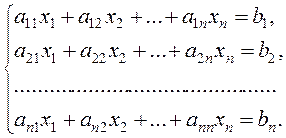(6)

Suppose that а11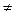0; let us divide the first equation by this coefficient:. (*)

Multiplying the resulting equation by –а21 and adding it to the second equation of system (6), we obtain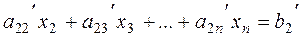.

Similarly, multiplying equation (*) by –аn1 and adding it to the last equation of system (6), we obtain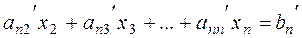.

At the end, we obtain the new system of equations with n1 unknowns: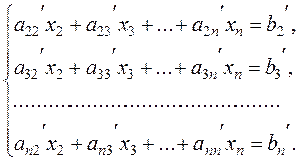(7)

System (7) is obtained from system (6) by applying linear transformations of equations; hence this system is equivalent to (6), i.e., any solution of system (7) is a solution of the initial system of equations.

To get rid of х2 in the third, the forth, …, nth-equation, we multiply the second equation of system (7) by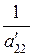and, multiplying this equation by the negative coefficients of х2 and summing them, obtainPerforming this procedure n times, we reduce the system of equations to the diagonal formWe determine хn from the last equation, substitute it in the preceding equation and obtain xn-1, and so on; going up, we determine х1 from the first equation. This is the classical Gauss method.

Consider the system of m equations with n unknowns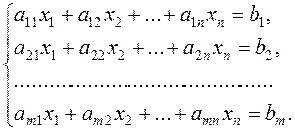(8)

Definition. The matrix composed of the coefficients of system (8) is called the principal matrix of this system: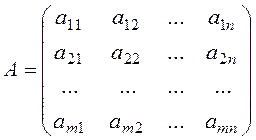.

Adding the column of free terms of system (8) to this matrix, we obtain the augmented matrix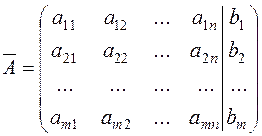.

The following linear operations on the rows of such a matrix are allowed:

- permutation of rows;

- multiplication of a row by some number and adding it to another row;

- permutation of columns (but we must remember to which unknowns they correspond);

- no operations on columns are allowed (columns cannot be multiplied by numbers, summed, etc).

The Gauss-Jordan method consists in reducing (by linear operation on rows) the principal matrix to the identity matrix, i.e., to the form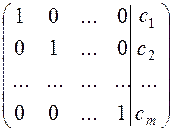.

If the columns were not interchanged, the solution of the system of linear equations is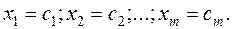Examples. Solve the following system of equations by the Gauss-Jordan method:We compose the augmented matrix of the system and, applying linear combinations of rows, reduce the principal matrix to the identity: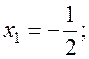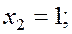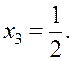Date: 2015-01-02; view: 1562

 <== previous page | next page ==> Matrices and Operations on Them | Rank of a Matrix
doclecture.net - lectures - 2014-2023 year. Copyright infringement or personal data (0.015 sec.)